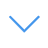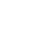# Math Game Multiple Choice

Math Game Multiple Choice is one of the math games that you can play on UGameZone.com for free. Be quick and choose the right answer for the shown equation. This game has a simple mechanism, but playing it is harder than you think! You are shown a simple equation (elementary arithmetic) and have a limited time to choose the right answer for it (there are 4 choices). Every correct answer earns you one score and your final score is based on the number of your correct answers.

Read Moreاضغط على 【Esc】 للخروج من وضع ملء الشاشة

# Math Game Multiple Choice

Math Game Multiple Choice is one of the math games that you can play on UGameZone.com for free. Be quick and choose the right answer for the shown equation. This game has a simple mechanism, but playing it is harder than you think! You are shown a simple equation (elementary arithmetic) and have a limited time to choose the right answer for it (there are 4 choices). Every correct answer earns you one score and your final score is based on the number of your correct answers.لتشغيل اللعبة ، تحتاج إلى السماح بتشغيل Adobe Flash Player في متصفحك.

You can use UGameZone Flash Enabler plugin to enable Flash automatically. Or you can follow our instructions to enable Flash manually.

play automatically

## Game Ratings

92%

يلعب 2030

تقييم 92%

الأصوات 917

917
434
Category
Math Games
الكلمات
وصف

Math Game Multiple Choice is one of the math games that you can play on UGameZone.com for free. Be quick and choose the right answer for the shown equation. This game has a simple mechanism, but playing it is harder than you think! You are shown a simple equation (elementary arithmetic) and have a limited time to choose the right answer for it (there are 4 choices). Every correct answer earns you one score and your final score is based on the number of your correct answers.

Step 1
Step 2